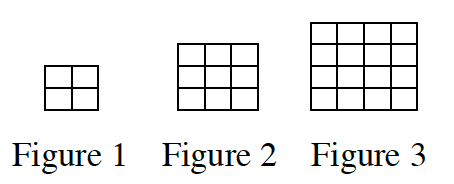### Home > CC3 > Chapter 2 > Lesson 2.1.7 > Problem2-69

2-69.

Examine the tile pattern below. Homework Help ✎1. On graph paper, draw Figures 4 and 5.

Figure 1 is a $2$-by-$2$ square
Figure 2 is a $3$-by-$3$ square
Figure 3 is a $4$-by-$4$ square.
That means Figure $x$ should be a $(x+1)$-by-$(x+1)$ square.

2. What would Figure 10 look like? How many tiles would it have? What about Figure 100?

Refer to the Hint in part (a).

Figure 10: An $11$-by-$11$ square that would have $121$ tiles.
Can you use the pattern to find what Figure 100 would look like?

3. Cami has a different tile pattern. She decided to represent the number of tiles of her pattern in a table, as shown below. Can you use the table to predict how many tiles would be in Figure 5 of her tile pattern? How many tiles would Figure 8 have? Explain how you know.

 Figure Number 1 2 3 4 Number of Tiles 5 9 13 17

Find the pattern in the chart.